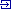What are you looking for?# SEVENPAR - Geo Software

From

SEVENPAR Geosoftware calculates from identical points in different geodetic Reference Systems exact parameter sets for the spatial Helmert / Bursa-Wolf Seven Parameter Transformation and the Molodensky Three Parameter Transformation.> The parameter sets can be used by GIS and transformation programs to compute Coordinate Transformations with accurate datum shifts between the Reference Systems.

## Software Details

SEVENPAR - Version 6.05 - Latest update on 1 August 2016
The latest important changes

1. Printing of the settings and the determined parameters.
2. WINDOWS©10 certification after 32Bit / 64Bit intensive test.
3. Calculation with up to 30.000 identical points.
4. More performance for parameter calculations with C++ functions from GeoDLL.
5. Calculation of a rotation matrix.

In many branches, custom defined areas and situations coordinates have to be transformed into different Coordinate Reference Systems (CRS). SEVENPAR is the tool of choice to calculate a Three or Seven Parameter Set to do coordinate transformations between that CRS. Considering that for both CRS each a list with coordinates of identical points are required. SEVENPAR is an very flexible tool for determining precisely Transformation Parameter Sets. Additional the program calculates a Rotation Matrix, the residuals of all identical points for further use, and as quality criterias the Maximum, Average and Mean Square (RMS) Residuals. The determined transformation parameters can be entered directly into suitable transformation software, e.g.TRANSDAT Coordinate Transformation, or into the calculation software of GPS receivers to do exact Coordinate Transformations.

## Detailed Program Description

Calculation of Parameter Sets for the Spatial Helmert and Molodensky Transformation with seven or three Parameters

The seven respectively three exact transformation parameters are computed with the aid of upto 30.000 identical points. Those are points with known coordinates in two different Reference Systems (geodetic datum). Suitable are Cartesian Coordinates and also Geographical Coordinates and UTM Coordinates with ellipsoidal height using the earth ellipsoid dimensions. Identical measuring points of higher order can be inquired or referred by the official measurement survays. The necessary ellipsoid half-axes and flattenings are available in the program for the selection from lists.

The coordinates of the identical points are tested intensively on outliers and deviations by different statistic procedures. The quality of the computed parameters is documented by a residual matrix and by the computation of the middle, maximum and root mean square (RMS) deviations.

The seven parameters of the Spatial Helmert Transformation are calculated in the three standards 'Coordinate Frame Rotation', 'Position Vector Transformation' and 'European Standard ISO 19111'. The three parameters for Molodenky parameter sets are calculated using the 'Standard Molodensky Transformation'.

The program has a user-selectable multi language interface and comes with extensive online help. The program can be run on most common networks and it is possible to use Terminal Services on WINDOWS Servers. In case of a network installation the clients are installed automatically by the program.

Calculation accuracy

All formulas used for the spatial Helmert transformation, the Molodensky transformation and for the statistic examinations are based on strict formulas. Approximations are not used.

There are to determine seven parameters of the spatial Helmert transformation, i.e. three translations, three rotations and a scale correction. The parameters are calculated by the compensation according to the method of the smallest squares (L2 norm, Helmert transformation). Therefore must be dissolved a formula system with seven equations and seven unknown quantities. The resulting parameters are calculated in the three most usual standards. Those are the Coordinate Frame Rotation, the Position Vector Transformation and the European Standard ISO 19111. Small-scale Helmert Parameter Sets, for example for an urban area, are very accurate and can be effective up to the centimeter range. Large-scale parameter sets, for example for an entire country, may well lie in the submeter range.

For the Molodensky transformation the program determines three parameters, i.e. three translations. The Molodensky transformation is one of the most widely used methods for transforming geodetic coordinates from one datum to another. The three parameters for Molodenky parameter sets are calculated using the Standard Molodensky Transformation. It is also one of the least accurate, due in large part to the fact that it does not account for rotation or scaling between datums. Errors on the order of meters are the norm with Molodensky transformations.

The coordinates of the identical points are tested intensively on outliers and deviations by different statistic procedures. The quality of the computed parameters is documented by a residual matrix and by the computation of the middle, maximum and root mean square (RMS) deviations.

The program SEVENPAR was tested with a reference program of the Geodetic Land Survey of the German state Mecklenburg-Vorpommern. The determined seven parameters of the spatial Helmert transformation exhibited only extremely slight deviations. With the translations dX, dY, dZ the deviation is less than 0,00001 meters; that corresponds the hundredth of a millimeter. With the rotations dRX, dRY, dRZ the deviation is less than 0,000001 seconds; that corresponds the millionth of a second. With the scale correction parameter dM the deviation is less than 0,000001 ppm.

Multilanguage support

The program SEVENPAR is equipped with a modern graphical user interface of which handling is to understand intuitively. For each window detailed help texts can be shown. The detailed electronic documentation of the program SEVENPAR is available in both English and German language. However, the user interface can be represented in many languages. For this purpose, external language files are used. When starting the program, it notes which language files are available in the calling directory. The found languages then are provided for selection in a list box. Bycreating new language files, new languages can be added to the program SEVENPAR at any time.

Network ability

The program SEVENPAR can be run on most common networks. The client installations works automatically. It is possible to use Terminal Services on WINDOWS Servers like e.g. 'Citrix Terminal Server'.

## Customer reviews

No reviews were found for SEVENPAR - Geo Software. Be the first to review!